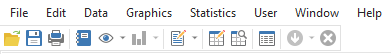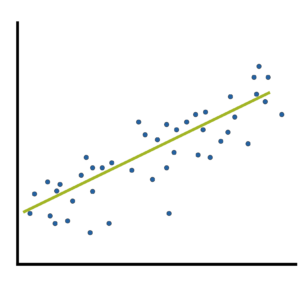# Stage 1

### Getting Started with Stata Tutorial #2: Stata Menus

August 11th, 2023 by

A great way to get started with Stata is using its menus.

The first part of this Tutorial Series introduced you to Stata’s windows.  You can now begin learning how to use Stata to work with data.

Across the top are 8 tabs: File, Edit, Data, Graphics, Statistics, User, Window, and Help.In our next article, we’ll look at two more: Graphics and Statistics.
(more…)

### Member Training: Operationalizing Research Questions

July 31st, 2023 byOne of the hardest steps in any project is learning to ask the right research question!
(more…)

### Get Started with Stata Tutorial #1: How Stata Windows Work

July 17th, 2023 by

So, you want to get started with Stata?Good choice!

At The Analysis Factor we recommend first becoming proficient in one statistical software. Then once you’ve progressed up to learning Stage 3 skills, adding a second statistical software. Whether it’s your first, second, or 5th statistical software, Stata has a lot that makes it worth learning.

When I first started using Stata, I remember being confused by the variety of menus and windows, the strange syntax of the code, the way it handled datasets… and what the heck is a do file? (more…)

### Two Types of Effect Size Statistic: Standardized and Unstandardized

June 26th, 2023 by

Effect size statistics are all the rage these days.

Journal editors are demanding them. Committees won’t pass dissertations without them.But the reason to compute them is not just that someone wants them — they can truly help you understand your data analysis.

### What Is an Effect Size Statistic?When many of us hear “Effect Size Statistic,” we immediately think we need one of a few statistics: Eta-squared, Cohen’s d, R-squared.
And yes, these definitely qualify. But the concept of an effect size statistic is actually much broader. Here’s a description from a nice article on effect size statistics:

“… information about the magnitude and direction of the difference between two groups or the relationship between two variables.

– Joseph A. Durlak, “How to Select, Calculate, and Interpret Effect Sizes”

If you think about it, many familiar statistics fit this description. Regression coefficients give information about the magnitude and direction of the relationship between two variables. So do correlation coefficients. (more…)

### The Effect Size: The Most Difficult Step in Calculating Sample Size Estimates

June 12th, 2023 by

One of the most difficult steps in calculating sample size estimates is determining the smallest scientifically meaningful effect size.Here’s the logic:

The power of every significance test is based on four things: the alpha level, the size of the effect, the amount of variation in the data, and the sample size.

You will measure the effect size in question differently, depending on which statistical test you’re performing. It could be a mean difference, a difference in proportions, a correlation, regression slope, odds ratio, etc.

When you’re planning a study and estimating the sample size needed for (more…)

### Member Training: Exact and Randomization Tests

June 2nd, 2023 byExact and randomization tests are simple from a conceptual level and need fewer assumptions than traditional parametric tests. They do require substantial computing power, but nothing that can’t be handled by the computer you have today. (more…)